# Sample Data: Urkiola Woods

Locations of birch and oak trees in a secondary wood in Urkiola Natural Park (Spain) annotated with birch / oak marks

## Details

Locations of birch and oak trees in a secondary wood in Urkiola Natural Park (Basque country, Spain) in a polygonal observation region bounded by the region Rectangle[{0.05, 0.05}, {219.95, 149.95}] meters, annotated with birch / oak marks.

## Examples

### Basic Examples (2)

Retrieve the data:

 In:=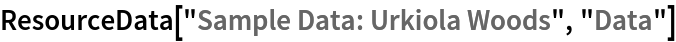Out=Summary of the spatial point data:

 In:=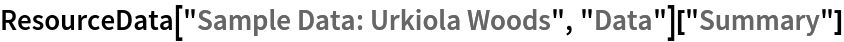Out=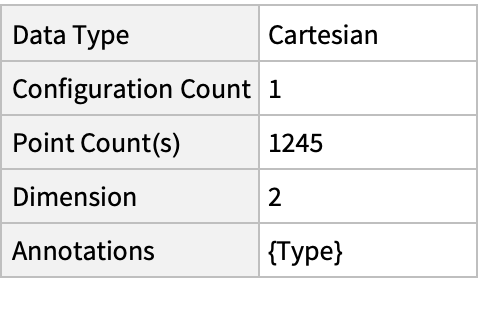### Visualizations (3)

Plot the spatial point data:

 In:=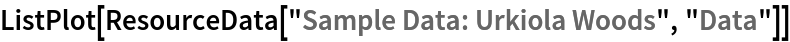Out=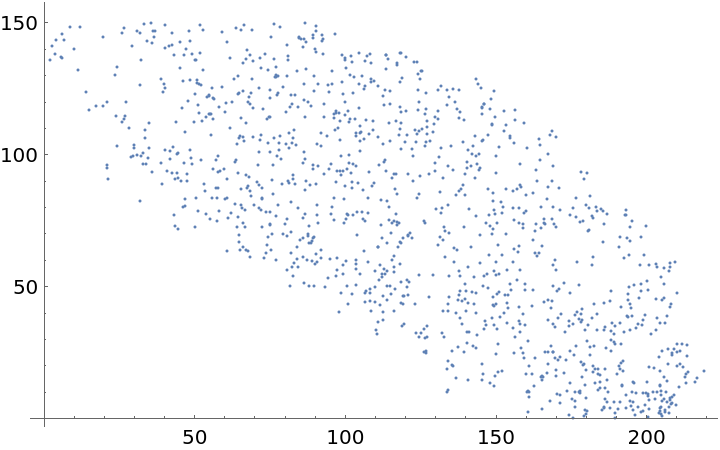Visualize the data with annotations:

 In:=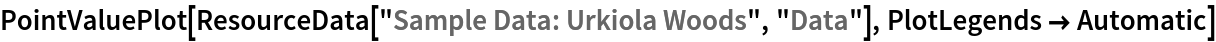Out=Visualize the smooth point intensity:

 In:=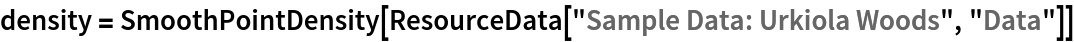Out=In:=Out=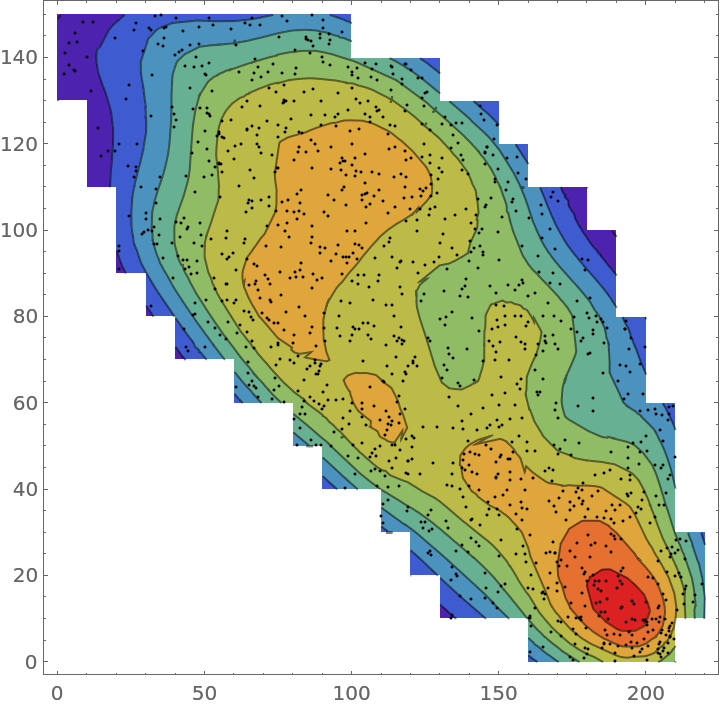### Analysis (3)

Compute probability of finding a point within given radius of an existing point - NearestNeighborG is the CDF of the nearest neighbor distribution:

 In:=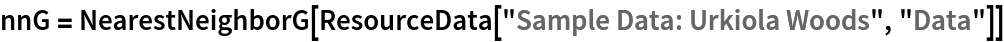Out=In:=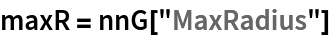Out=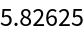In:=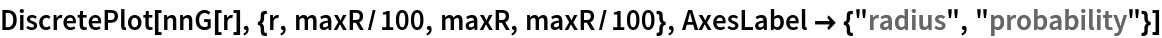Out=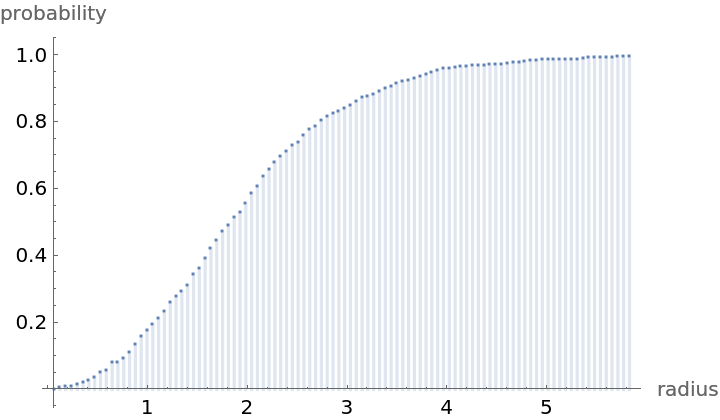Mean distance between a typical point and its nearest neighbor (for positive support distribution can be approximated via a Riemann sum of 1-CDF):

 In:=In:=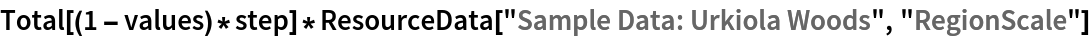Out=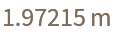Test for complete spatial randomness:

 In:=Out=Gosia Konwerska, "Sample Data: Urkiola Woods" from the Wolfram Data Repository (2021)